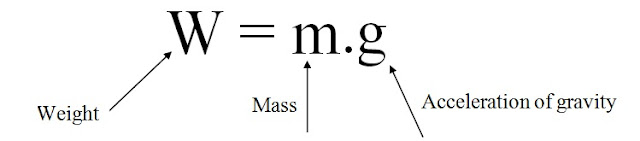Sometimes much confusion and misunderstanding is created, while using the various systems of units in the measurement of force and mass. This happens because of the lack of clear understanding of the difference between mass and weight. The following definitions of mass and weight should be clearly understood.
• Mass
It is the amount of matter contained in a given body and does not vary with the change in its position on the earth's surface. The mass of a body is measured by direct comparison with a standard mass by using a lever balance.
• Weight
It is the amount of pull, which the earth exerts upon a given body. Since  the pull varies with the distance of the body from the center of the earth. Therefore weight of the body will also vary with its position on the earth's surface (say latitude and elevation). It is thus obvious that, the weight is a force.

The weight of an object is defined as the force of gravity on the object and may be calculated as the  mass times the acceleration of gravity. Since the weight is a force, its SI unit is the newton.